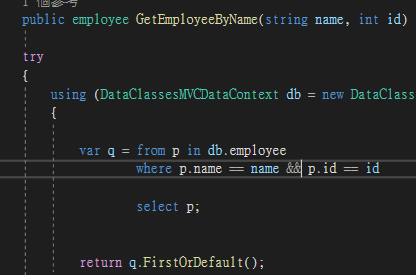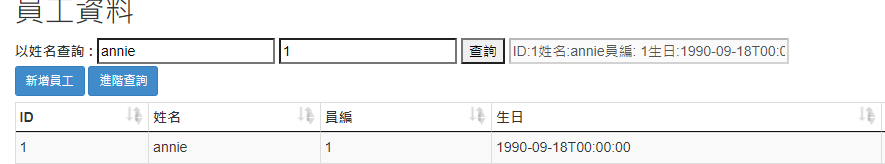#0

## Linq to SQL 問題

``````var q = from p in db.employee
where p.name == name || p.id == id
select p;
``````### 4 個回答

0

``````var q = from p in db.employee
where (p.name == name || string.IsNullOrEmpty(name)) &&
(p.id == id || string.IsNullOrEmpty(id))
select p;
``````

0

``````var q = from p in db.employee
where p.name.Contains(name.Trim()) || p.id == id
select p;
``````

0

``````var q = p.Where(x => x.ip == ip)
``````

0